# 固定批次的稳定性研究的均值响应的保质期的方法和公式

## 只有一个规格下限的均值响应方法的保质期

I = 批次水平总数

n = 响应值总数

X = 模型的设计矩阵

I = 批次水平总数

n = 响应值总数

X = 模型的设计矩阵

### 具有时间的模型

I = 批次水平总数

n = 响应值总数

X = 模型的设计矩阵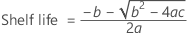### 表示法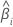i 个批次的斜率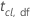置信区间 (cl) 和自由度 (df) 的 t 统计量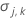估计参数向量的方差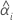i 个批次的截距
L规格下限
X设计矩阵
ii表示保质期估计值所在的批次的指数
l批次因子中的水平数
yiji 个批次和第 j 个时间的响应值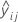i 个批次和第 j 个时间的拟合值
n响应值总数

## 只有一个规格上限的均值响应方法的保质期

I = 批次水平总数

n = 响应值总数

X = 模型的设计矩阵

I = 批次水平总数

n = 响应值总数

X = 模型的设计矩阵

I = 批次水平总数

n = 响应值总数

X = 模型的设计矩阵

### 表示法i 个批次的斜率置信区间 (cl) 和自由度 (df) 的 t 统计量估计参数向量的方差i 个批次的截距
U规格上限
X设计矩阵
ii表示保质期估计值所在的批次的指数
l批次因子中的水平数
yiji 个批次和第 j 个时间的响应值i 个批次和第 j 个时间的拟合值
n响应值总数

## 两种规格限

I = 批次水平总数

n = 响应值总数

X = 模型的设计矩阵

I = 批次水平总数

n = 响应值总数

X = 模型的设计矩阵

I = 批次水平总数

n = 响应值总数

X = 模型的设计矩阵

### 表示法i 个批次的斜率
l批次因子中的水平数
n数据中的行数具有自由度 (DF) 的 t 分布的逆累积分布函数在 cl 处的值